# Basic Algebra Videos

Stop wasting time on Youtube. Browse our curated collection of amazing Basic Algebra videos. We did all the work for you!### A Different Way to Solve Quadratic Equations

• 0
• 0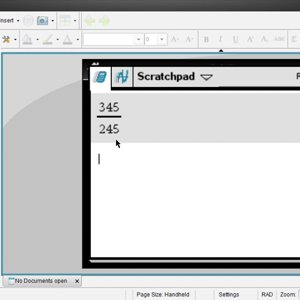### TI Nspire™ CX CAS Tutorial - Basic Fraction Operations

• 0
• 0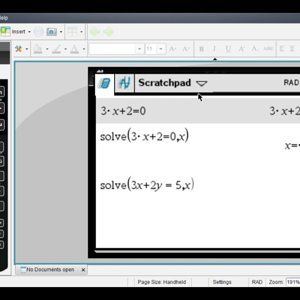### TI Nspire™ CX CAS Tutorial - Solving Basic Equations - YouTube

• 0
• 0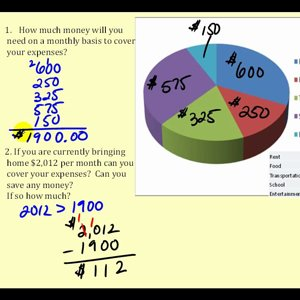### Problem Solving: Adding and Subtracting Whole Numbers

• 0
• 1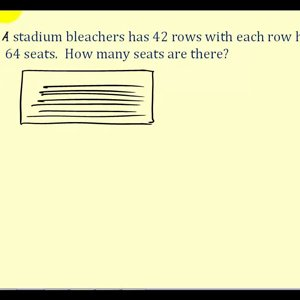### Problem Solving Using Whole Number Operations

• 0
• 0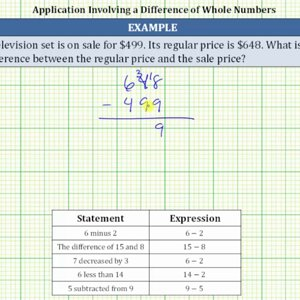### Find the Difference of an Original and Sale Price (Whole Numbers)

• 0
• 0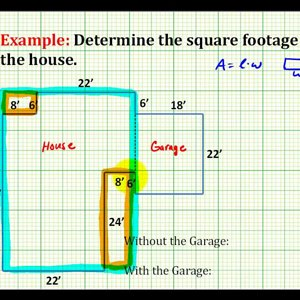### Example: Determining the Square Footage of a House

• 0
• 0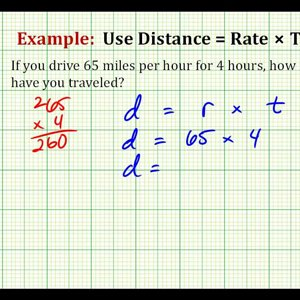### Example 1: Solve a Problem using Distance = Rate x Time

• 0
• 0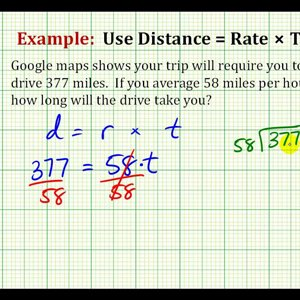### Example 2: Solve a Problem using Distance = Rate x Time

• 0
• 0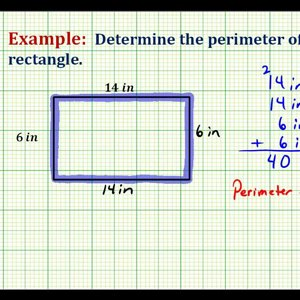### Ex: Determine the Perimeter of a Rectangle Involving Whole Numbers

• 0
• 0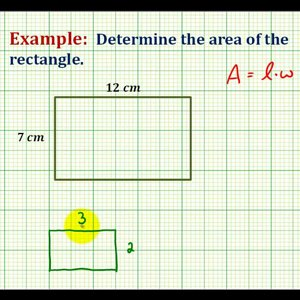### Ex: Determine the Area of a Rectangle Involving Whole Numbers

• 0
• 0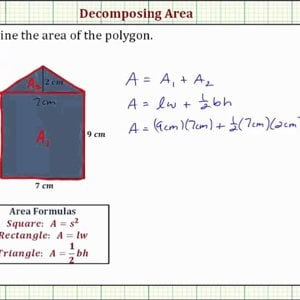### Ex: Determine the Area of a Pentagon By Decomposing the Area (Rect + Tri)

• 0
• 0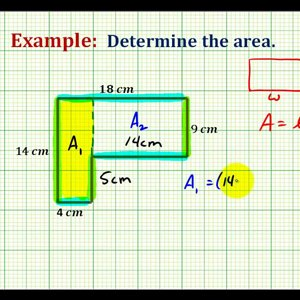### Ex: Find the Area of an L-Shaped Polygon Involving Whole Numbers

• 0
• 0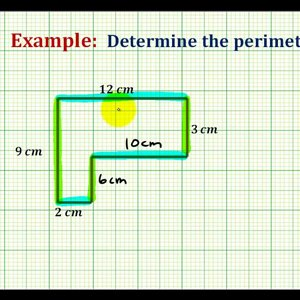### Ex: Find the Perimeter of an L-shaped Polygon Involving Whole Numbers

• 0
• 0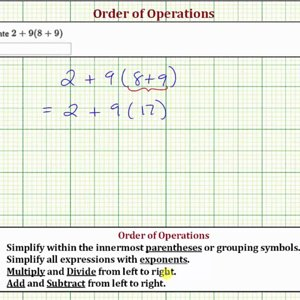### Evaluate an Expression Using the Order of Operations: a+b(c+d)

• 0
• 0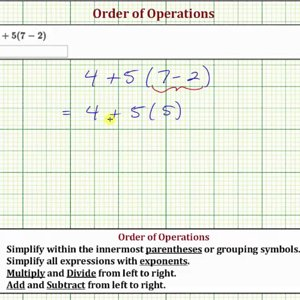### Evaluate an Expression Using the Order of Operations: a+b(c-d)

• 0
• 0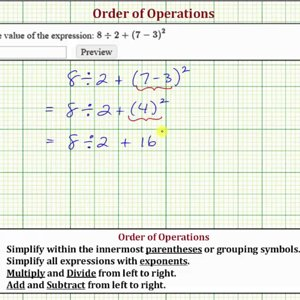### Evaluate an Expression Using the Order of Operations: a/b+(c-d)^2

• 0
• 0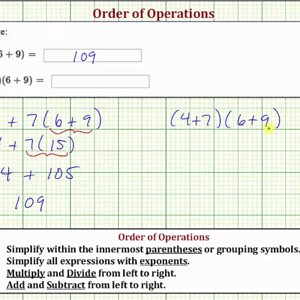### Evaluate an Expression Using the Order of Operations: a+b(c+d), (a+b)(c+d)

• 0
• 0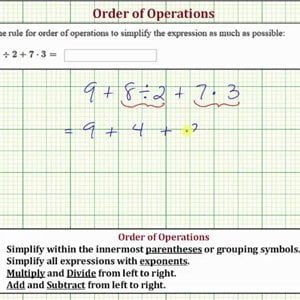### Evaluate an Expression Using the Order of Operations: a+b/c+d*f

• 0
• 0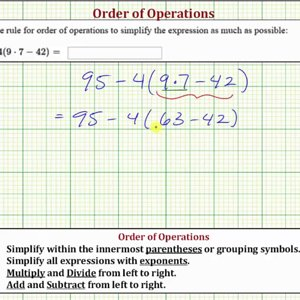### Evaluate an Expression Using the Order of Operations: a-b(c*d-e)

• 0
• 0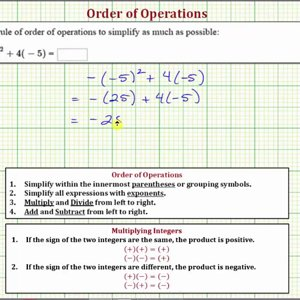### Ex 1: Evaluate an Expression using Order of Operations: a^2-bcd (integers)

• 0
• 0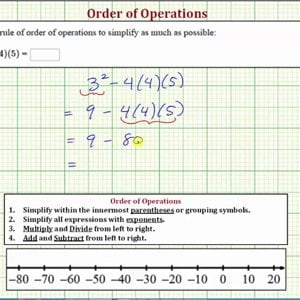### Ex 2: Evaluate an Expression using Order of Operations: a^2-bcd (integers)

• 0
• 0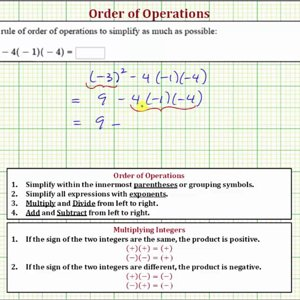### Ex 3: Evaluate an Expression using Order of Operations: a^2-bcd (integers)

• 0
• 0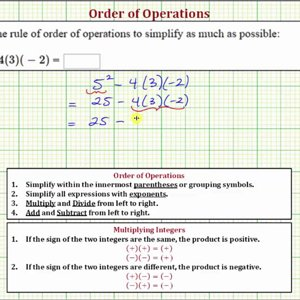### Ex 4: Evaluate an Expression using Order of Operations: a^2-bcd (integers)

• 0
• 0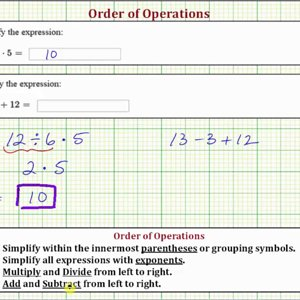### Ex: Evaluate an Expressions Using Order of Operations: a/b*c , a-b+c (integers)

• 0
• 0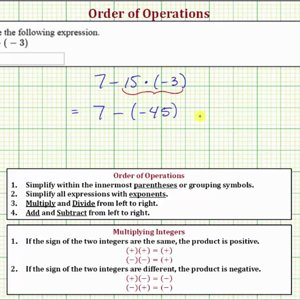### Ex: Evaluate an Expression Using Order of Operations: a-b*c (integers)

• 0
• 0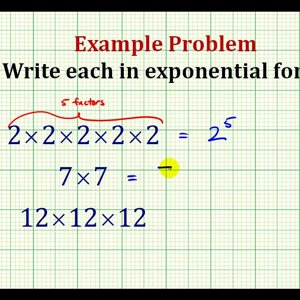### Example: Write Repeated Multiplication in Exponential Form

• 0
• 0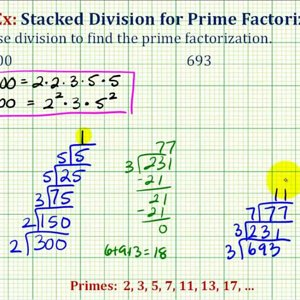### Ex 1: Prime Factorization Using Stacked Division

• 0
• 0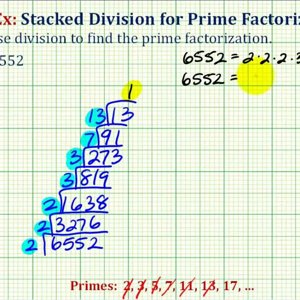### Ex 2: Prime Factorization Using Stacked Division

• 0
• 0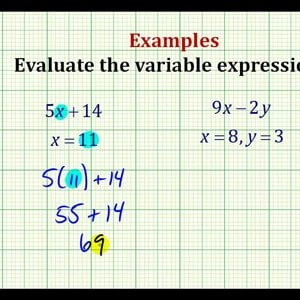### Examples Part 1: Evaluating Variable Expressions

• 0
• 0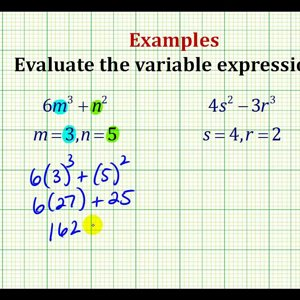### Examples Part 2: Evaluating Variable Expressions

• 0
• 0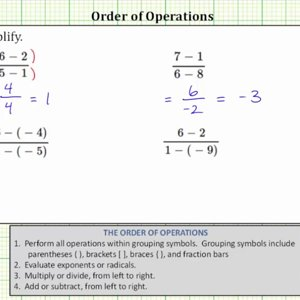### Simplify Basic Expressions in Fraction Form

• 0
• 0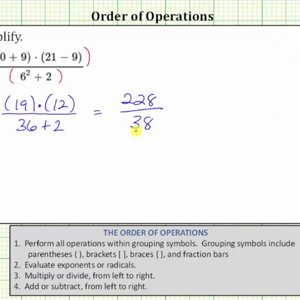### Ex 1: Simplify an Expression in Fraction form (Order of Operations)

• 0
• 0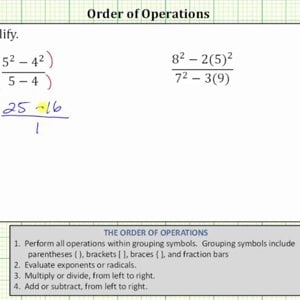### Ex 2: Simplify an Expression in Fraction form (Order of Operations)

• 0
• 0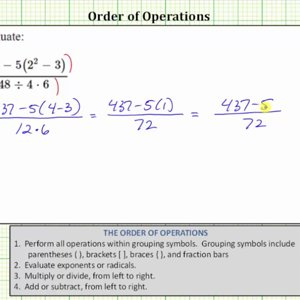### Ex 3: Simplify an Expression in Fraction form (Order of Operations)

• 0
• 0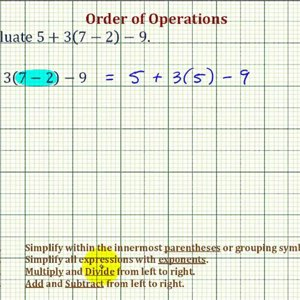### Ex: Evaluate an Expressing Using the Order of Operations

• 0
• 0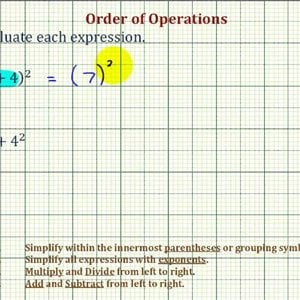### Ex: Evaluate Expressions - Square of a Sum and the Sum of Squares

• 0
• 0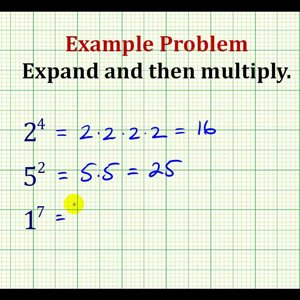### Examples: Evaluate Exponential Expressions

• 0
• 0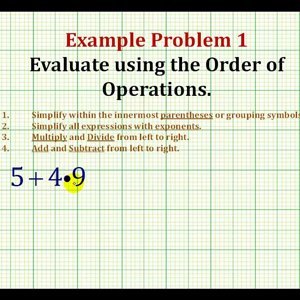### Example 1: Evaluate An Expression Using The Order of Operations

• 0
• 0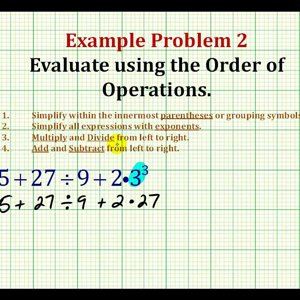### Example 2: Evaluate An Expression Using The Order of Operation

• 0
• 0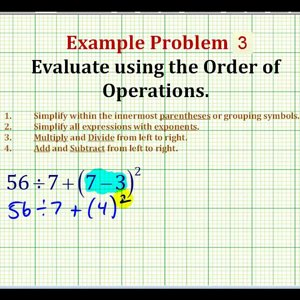### Example 3: Evaluate An Expression Using The Order of Operation

• 0
• 0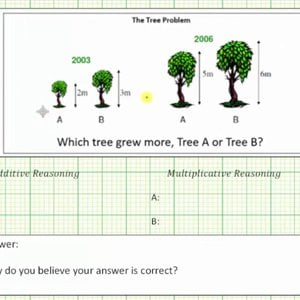### Additive and Multiplicative Reason - The Tree Problem

• 0
• 0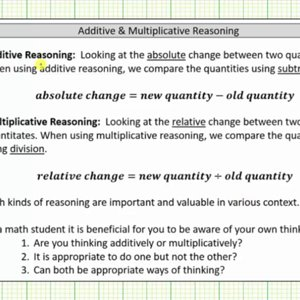### Additive and Multiplicative Reason - The Broomstick Problem

• 0
• 0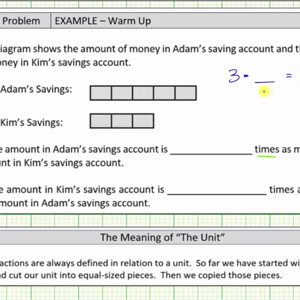### Multiplicative Reasoning - Comparing Savings Accounts

• 0
• 0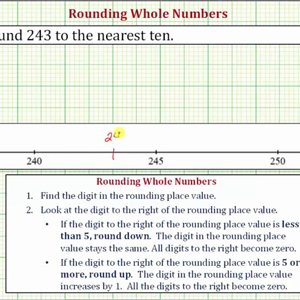### Ex: Rounding to the Nearest Ten - Number Line / Formal Rules

• 0
• 0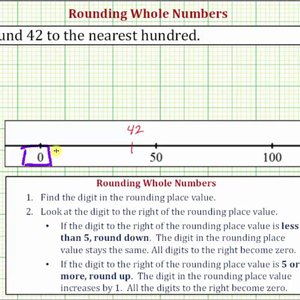### Ex: Rounding to the Nearest Hundred - Number Line / Formal Rules

• 0
• 0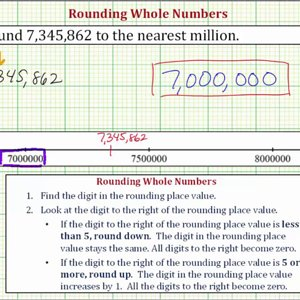### Ex: Rounding to the Various Place Values - Number Line / Formal Rules

• 0
• 0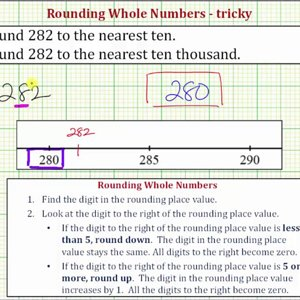### Ex: Round a 3 Digit Number to Tens and Ten Thousands (tricky)

• 0
• 0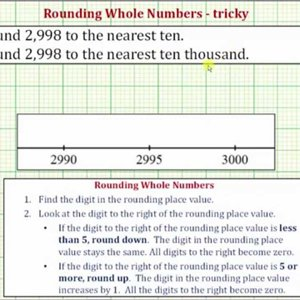### Ex: Round a 4 Digit Number to Tens and Ten Thousands (tricky)

• 0
• 0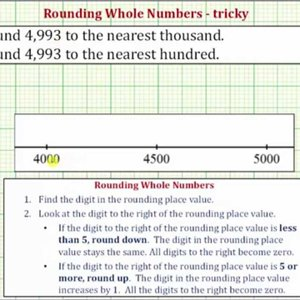### Ex: Round a 4 Digit Number to Hundreds and Thousands (tricky)

• 0
• 0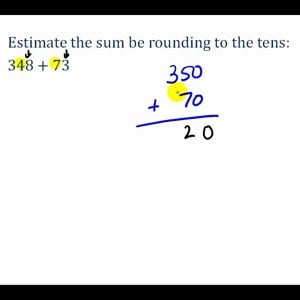### Estimating Addition and Subtraction Problems Involving Whole Numbers

• 0
• 0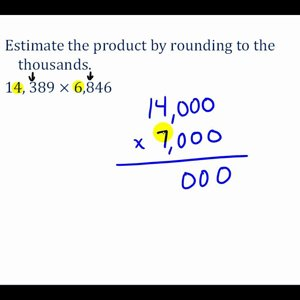### Estimating Multiplication and Division Problems Involving Whole Numbers

• 0
• 0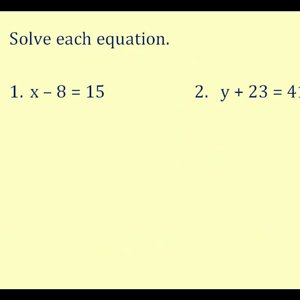### Solving Equations by Addition and Subtraction

• 0
• 3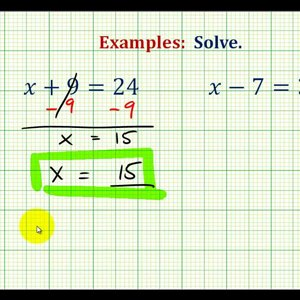### Ex: Solve One Step Equations By Adding and Subtracting Whole Numbers (Var. on Left)

• 0
• 0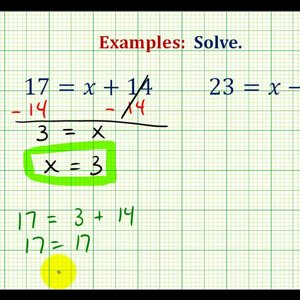### Ex: Solve One Step Equations By Adding and Subtracting Whole Numbers (Var on Right)

• 0
• 0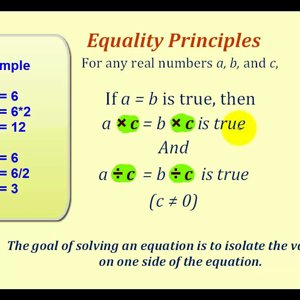### Solving Equations by Multiplying and Dividing

• 0
• 0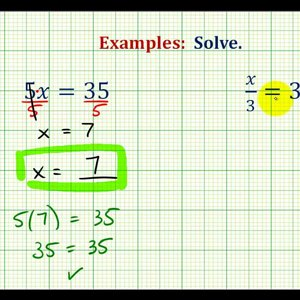### Ex: Solve One Step Equations By Multiplying and Dividing Whole Numbers (Var. on Left)

• 0
• 0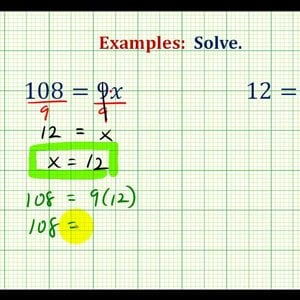### Ex: Solve One Step Equations By Multiplying and Dividing Whole Numbers(Var on Right)

• 0
• 0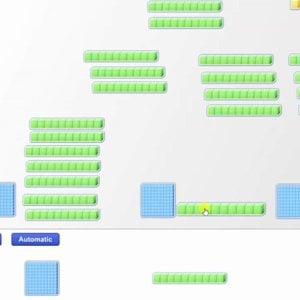### Partitive Division with Base 10 Blocks: 3 Digit Divided by 1 Digit with No Remainder

• 0
• 0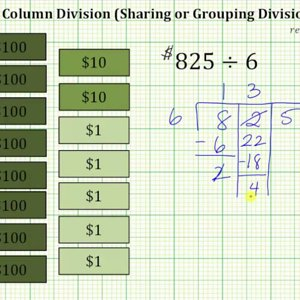### Column Divisions: 3 Digit Divided by 1 Digit with Remainder

• 0
• 0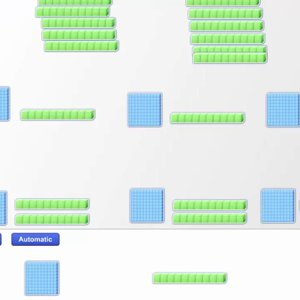### Partitive Division with Base 10 Blocks: 3 Digit Divided by 1 Digit with Remainder

• 0
• 0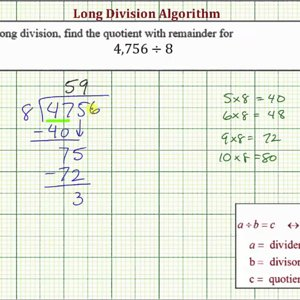### Ex: Long Division - Four Digit Divided by One Digit (With Remainder)

• 0
• 0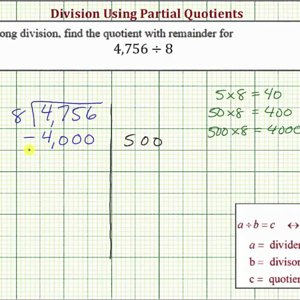### Ex: Division Using Partial Quotient - Four Digit Divided by One Digit (With Remainder)

• 0
• 0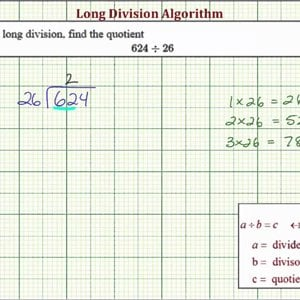### Ex: Long Division - 3 Digit Divided by 2 Digit (No Remainder)

• 0
• 0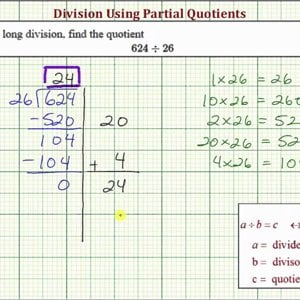### Ex: Division Using Partial Quotient - 3 Digit Divided by 2 Digit (No Remainder)

• 0
• 0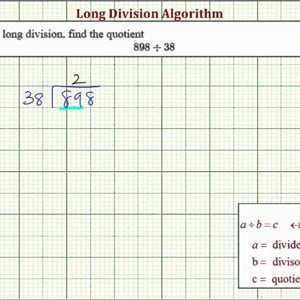### Ex: Long Division - 3 Digit Divided by 2 Digit (With Remainder)

• 0
• 0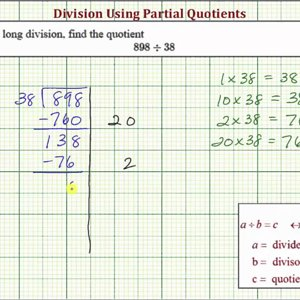### Ex: Division Using Partial Quotient - 3 Digit Divided by 2 Digit (With Remainder)

• 0
• 0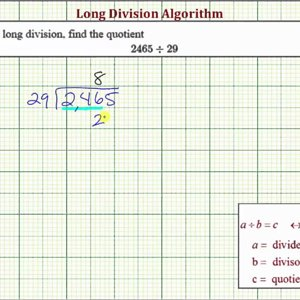### Ex: Long Division - 4 Digit Divided by 2 Digit (No Remainder)

• 0
• 0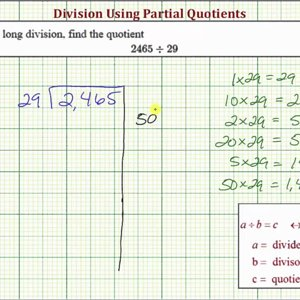### Ex: Division Using Partial Quotient - 4 Digit Divided by 2 Digit (No Remainder)

• 0
• 0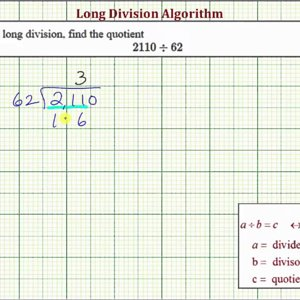### Ex: Long Division - 4 Digit Divided by 2 Digit (With Remainder)

• 0
• 0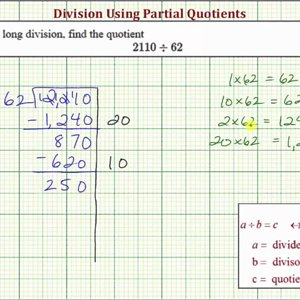### Ex: Division Using Partial Quotient - Four Digit Divided by One Digit (With Remainder)

• 0
• 0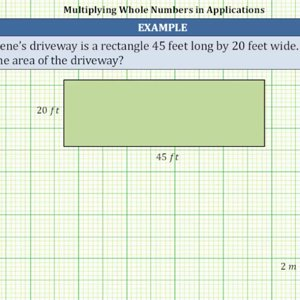### >Multiply Whole Numbers to Solve Applications

• 0
• 0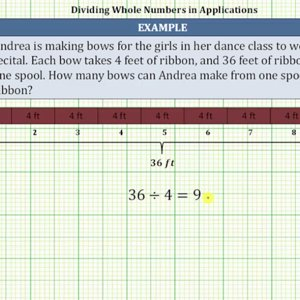### Divide Whole Numbers to Solve Applications

• 0
• 0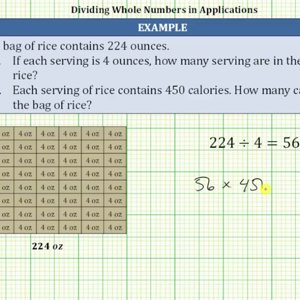### Divide and Multiply Whole Numbers to Solve Applications

• 0
• 0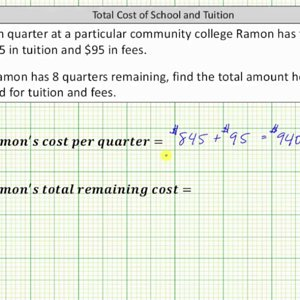### Total Cost using Addition and Multiplication

• 0
• 0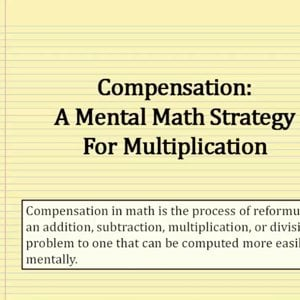### Mental Math: Multiplication Using Compensation

• 0
• 0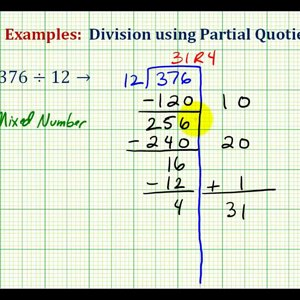### Partial Quotients - Dividing Whole Numbers

• 0
• 0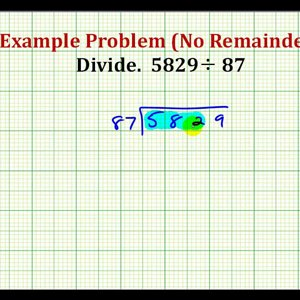### Example: Dividing Whole Numbers without a Remainder

• 0
• 0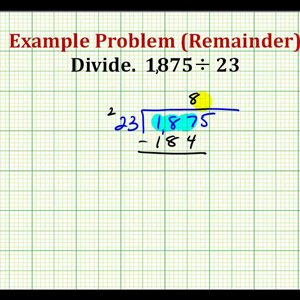### Example: Dividing Whole Numbers with a Remainder

• 0
• 0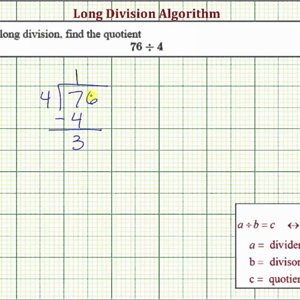• 0
• 0